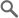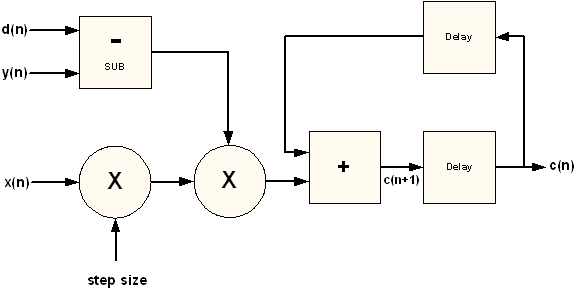# LMS (Least Mean Square) Adaptive Filter

#### Related ProductsAdaptive algorithms are a mainstay of Digital Signal Processing (DSP). They are used in a variety of applications including acoustic echo cancellation, radar guidance systems, and wireless channel estimation, among many others.

An adapative algorithm is used to estimate a time varying signal. There are many adaptive algorithms such as Recursive Least Square (RLS) and Kalman filters, but the most commonly used is the Least Mean Square (LMS) algorithm. It is a simple but powerful algorithm that can be implemented to take advantage of Lattice FPGA architectures. Developed by Window and Hoff, the algorithm uses a gradient descent to estimate a time varying signal. The gradient descent method finds a minimum, if it exists, by taking steps in the direction negative of the gradient. It does so by adjusting the filter coefficients to minimize the error.

The LMS reference design consists of two main functional blocks - a FIR filter and the LMS algorithm. The FIR filter is implemented serially using a multiplier and an adder with feedback. The FIR result is normalized to minimize saturation. The LMS algorithm iteratively updates the coefficient and feeds it to the FIR filter. The FIR filter than uses the coefficient c(n) along with the input reference signal x(n) to generate the output y(n). The output y(n) is then subtracted to from the desired signal d(n) to generate an error, which is used by the LMS algorithm to compute the next set of coefficients.

### Features

• Input data bit width
• Output data bit width
• Binary point
• Tap size
• Step size

### Block Diagram### Performance and Size

Results for LatticeECP1
Configuration Language SLICEs LUTs Mult 36x36 Mult18x18 sysMEM EBRs fMAX(MHz)
Input data bit width =16,
output data bit width = 24,
binary point = 13,
tap size = 64,
step size - initial simulation with u=0.2,
and then with u=1.0
Verilog 204 209 3 1 3 76

Note: The performance and design sizes shown above are estimates only for LatticeECP33E-5F672C. The actual results may vary depending upon the chosen parameters, timing constraints, and device implementation. See the design's documentation for details. All coding and design work was done on a PC platform unless noted otherwise.

Note: The performance and design sizes shown above are estimates only for LatticeECP33E-5F672C. The actual results may vary depending upon the chosen parameters, timing constraints, and device implementation. See the design's documentation for details. All coding and design work was done on a PC platform unless noted otherwise.

### Documentation

 Technical Resources
TITLE NUMBER VERSION DATE FORMAT SIZE